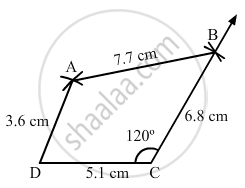Share

# Construct a Quadrilateral Abcd in Which Ab = 7.7 Cm, Bc = 6.8 Cm, Cd = 5.1 Cm, Ad = 3.6 Cm and ∠C = 120°. - Mathematics

Course
ConceptConstructing a Quadrilateral When Two Adjacent Sides and Three Angles Are Known

#### Question

Construct a quadrilateral ABCD in which AB = 7.7 cm, BC = 6.8 cm, CD = 5.1 cm, AD = 3.6 cm and ∠C = 120°.

#### Solution

Steps of construction:

Step I: Draw DC = 5 . 1 cm .

$\text { Step II: Construct} \angle DCB = 120^\circ .$

Step III : With C as the centre and radius 6 . 8 cm, cut off BC = 6 . 8 cm .

Step IV: With B as the centre and radius 7 . 7 cm, draw an arc .

Step V : With D as the centre and radius 3 . 6 cm, draw an arc to intersect the arc drawn in Step IV at A .

Step VI: Join AB and AD to obtained the required quadrilateral .Is there an error in this question or solution?

#### APPEARS IN

RD Sharma Solution for Mathematics for Class 8 by R D Sharma (2019-2020 Session) (2017 to Current)
Chapter 18: Practical Geometry (Constructions)
Ex. 18.3 | Q: 3 | Page no. 8
Solution Construct a Quadrilateral Abcd in Which Ab = 7.7 Cm, Bc = 6.8 Cm, Cd = 5.1 Cm, Ad = 3.6 Cm and ∠C = 120°. Concept: Constructing a Quadrilateral - When Two Adjacent Sides and Three Angles Are Known.
S